## Explaining ML with neural networks

I’m working on interactive visualisations for Machine Learning for Kids that explain more of the machine learning models that children create.

Machine Learning for Kids is a platform to teach children about artificial intelligence and machine learning, by giving them a simple tool for training machine learning models, and using that to make projects using tools like Scratch. I’ve described before how I’ve seen children learn a lot about machine learning principles by being able to play and experiment with it.

But I still want the site to do more to explain how the tech actually works. I’ve done this before for the decision tree classifiers that students train for numbers projects but with this new feature I’m trying to explain neural networks.

I’ve recorded a video run-through of what I’ve done so far. The screenshots below link to different sections of the video.

For this demo, I’m going to use a project based on smart assistants – where students train a model to understand a few different commands. e.g. If they give it a command to turn on the fan, their model has been trained to recognise that and the fan starts spinning.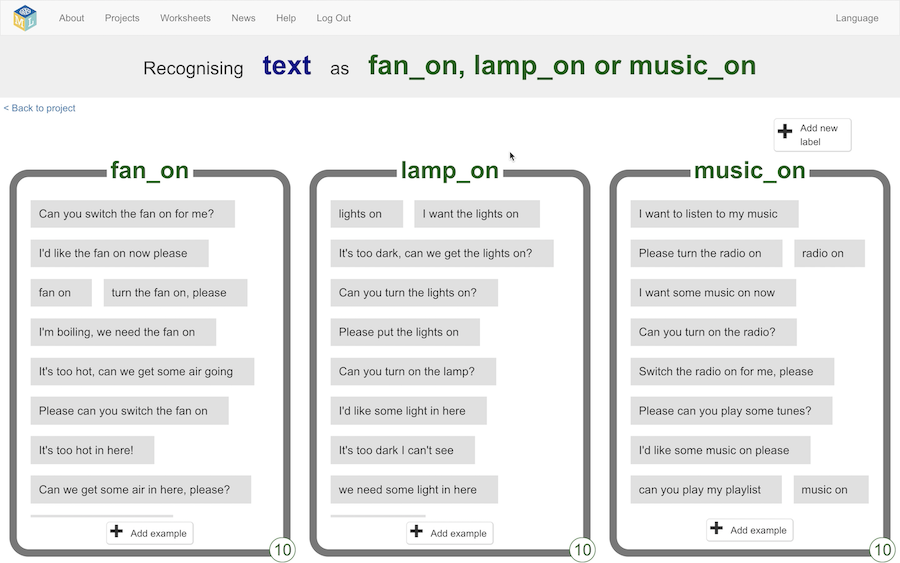They train this by creating these three buckets, filling them with examples of how to give each of the commands, and using these training examples to create their own custom machine learning model.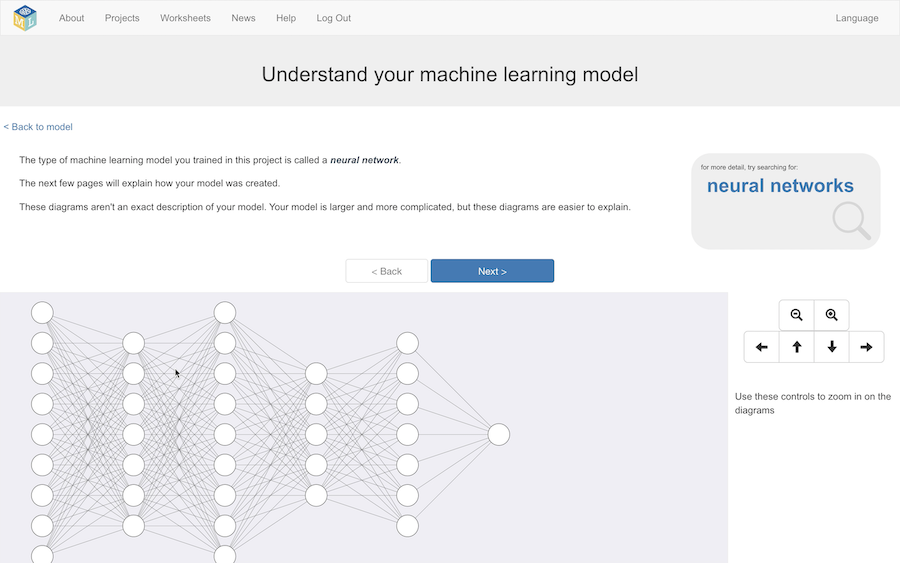This is the new section.

My goal is to use this to give students an introduction to how the neural network was trained for their project.

It’s not an accurate visualisation of their actual model, because that would be too complicated – so I’m showing them a visualisation that has enough elements in common with their actual model to be able to explain the idea.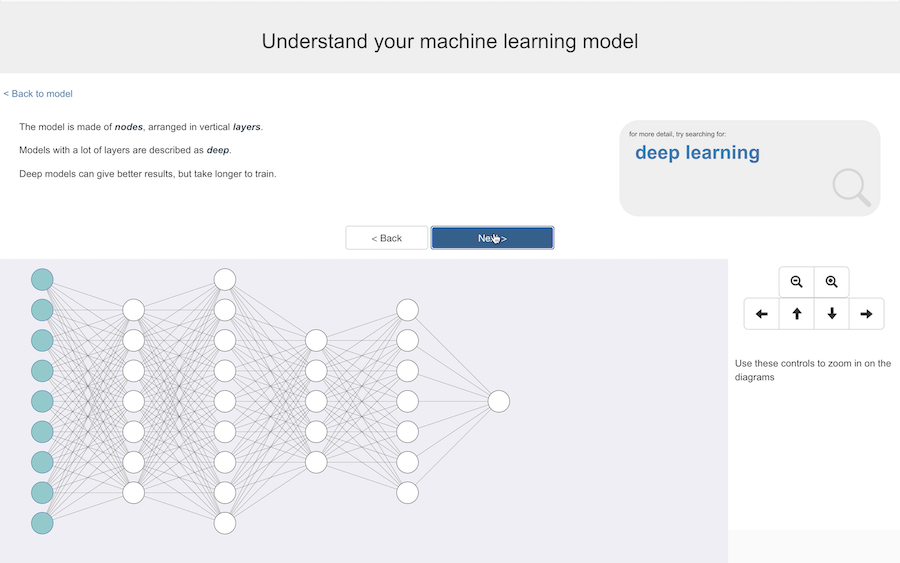To start with, I use a very traditional neural network diagram, to explain how the model is made up of these nodes shown as circles, that are arranged in layers.

And that models with a lot of these layers are described as deep – which can give good results, but can take a long time to train.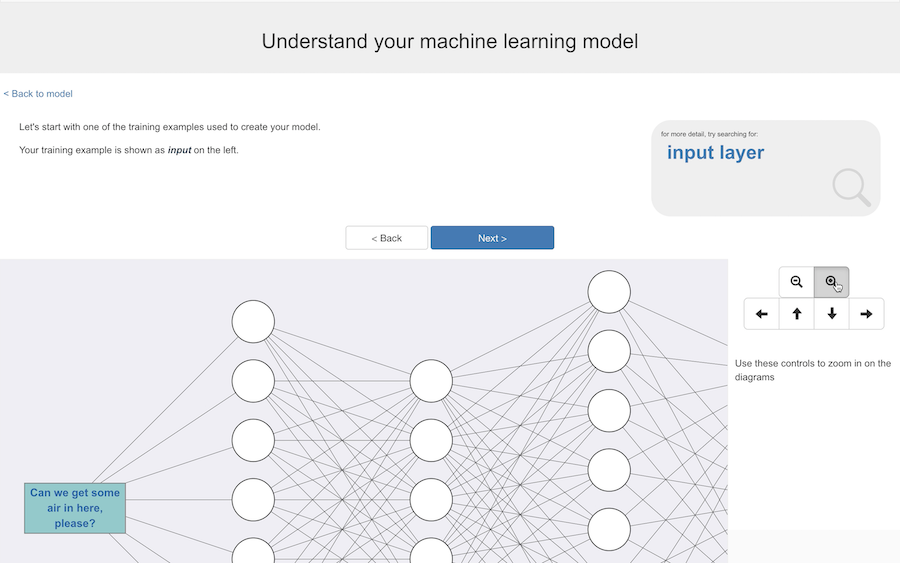Now I start stepping them through the model.

Instead of doing it in the abstract, I do it with one of their training examples – this is something they will have typed into one of their training buckets.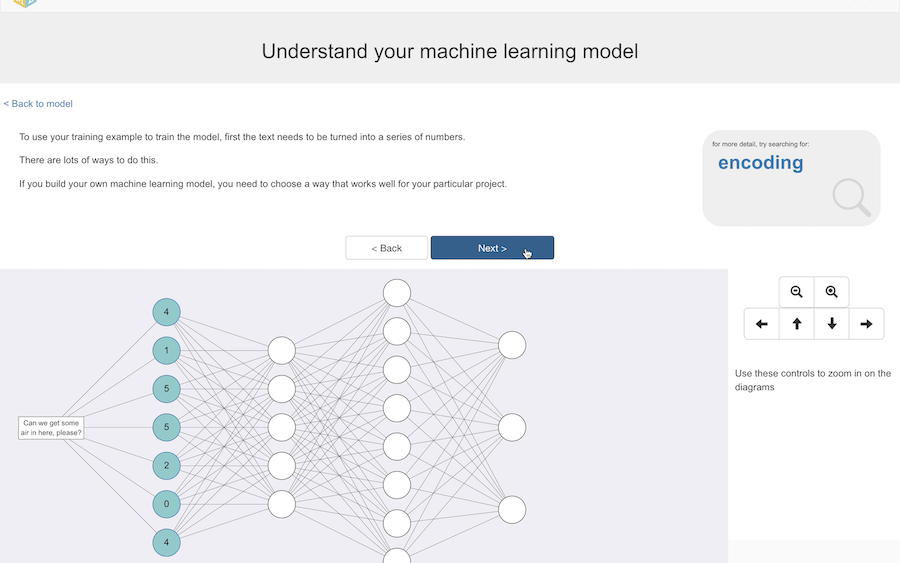To use it to train the model, first this text needs to be turned into a set of numbers.

There are lots of different ways to do this, and if you build your own model you need to choose a method that is a good fit for your project.

This site is used by children spanning a wide range of ages, so I’m trying to strike a balance in how I explain things.

I’ve gone with two sections at the top of the page.

On the left, I try to explain the ideas as simply as I can, without using jargon or technical terms.

On the right, I give the technical term for what this page is about – so older children who can handle a little more detail get a jumping off point for more background reading. (And the text in this jargon panel for each page is a customized link to help them get started finding out more)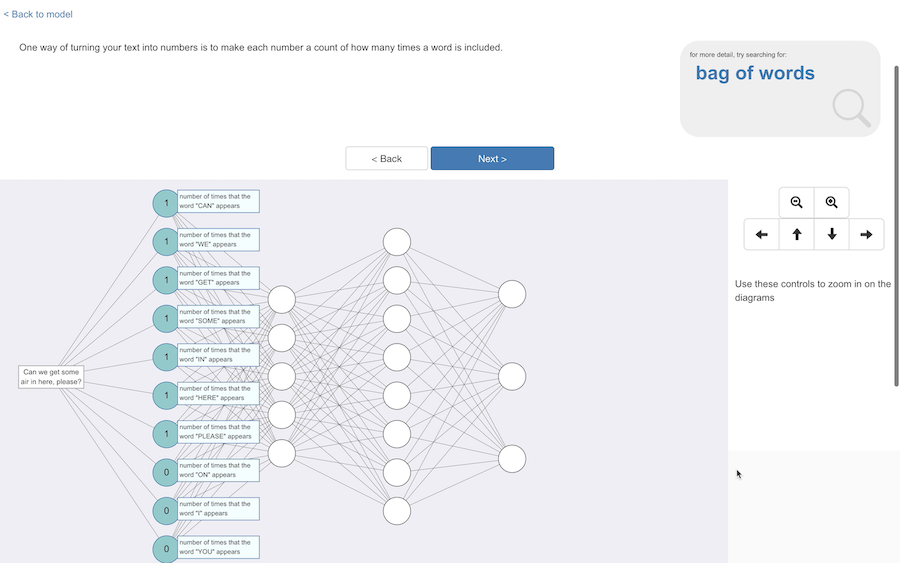The first step is turning their input text into numbers, and there are lots of ways to do that.

One way is to count how many times each word shows up.

I show them an example of that – not an abstract hard-coded visualisation but using the most common words across all of their training examples, so they hopefully recognize having typed these words in most frequently.

And for students who want to know more, more information about this technique – bag of words – can be found from the jargon panel in the top-right.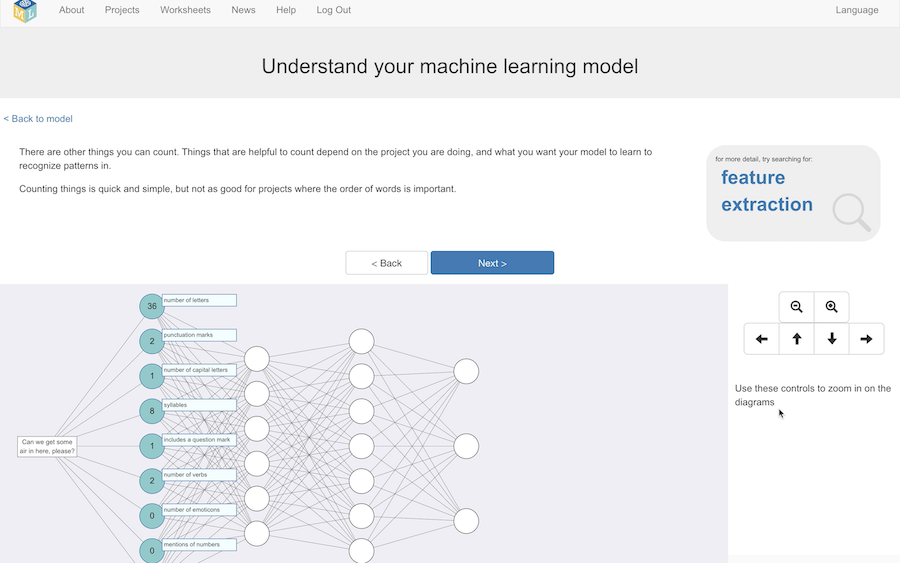There are other things you can count.

I show them a few trivial examples, like the number of letters, the use of punctuation, or the use of contractions or emoticons.

What is actually useful to count depends on the type of project you’re doing, as these features are what the model is going to be able to learn to recognize patterns in.

But there are limitations of doing this, because you’re not giving the model the chance to learn anything about the order of words.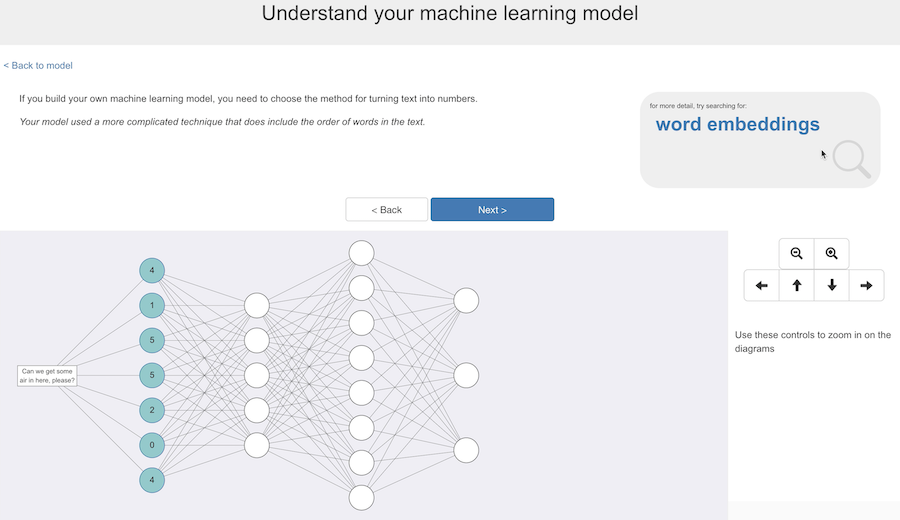The technique used for their actual model does use vectors to represent sequences of words, so the order of words is captured for their project.

I think that explaining how that works is a bit complex to get into, so I leave the students a link to word embeddings if they want to find out more but otherwise leave it there.

The important point for them to understand is that the first thing that happens is the input text is turned into a series of numbers.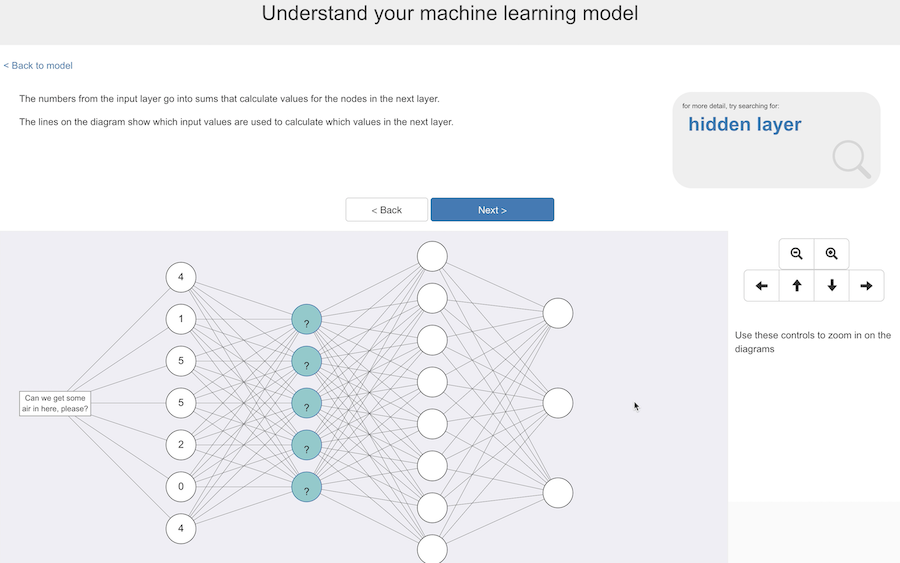Next, these numbers are used in sums that calculate values for nodes in the next layer along.

And the connecting lines show which input values contribute to each of these nodes in the next layer.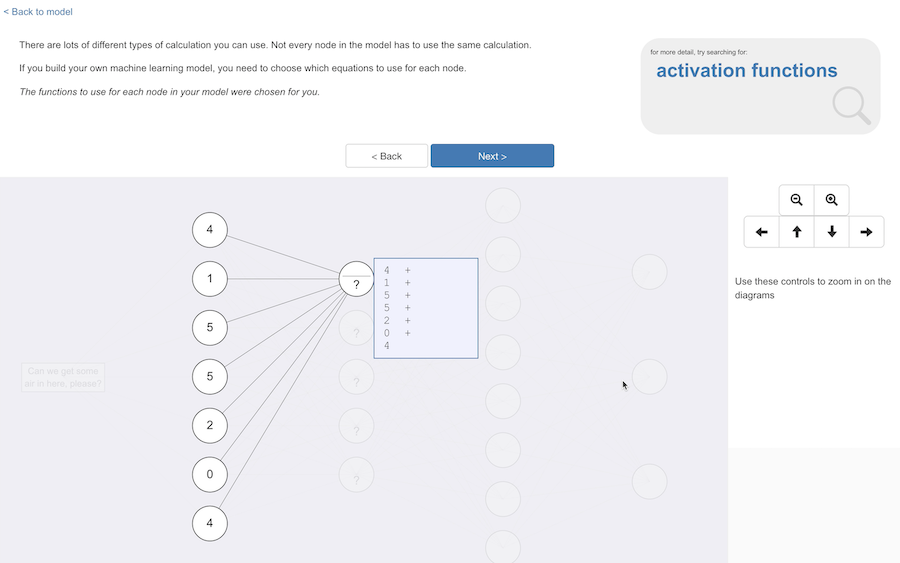There are lots of different type of sum that you can use for this.

(And not every node in the model has to use the same type of calculation. If you build your own machine learning model, you have to choose the functions to use for each node.)

You can see here the values from each of the input nodes, in a starting point for the sort of sum you can use.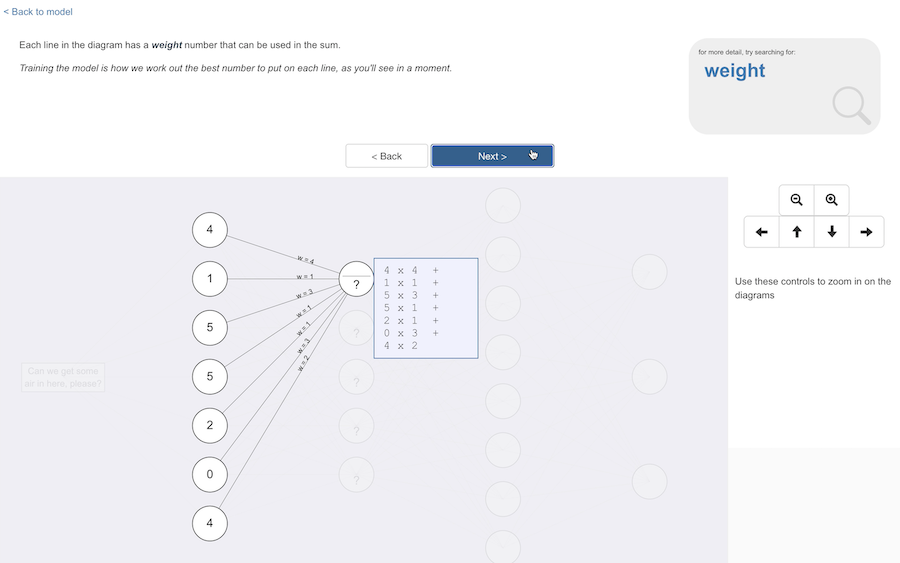Each connecting line in the diagram has a number associated with it that can be used in the sum.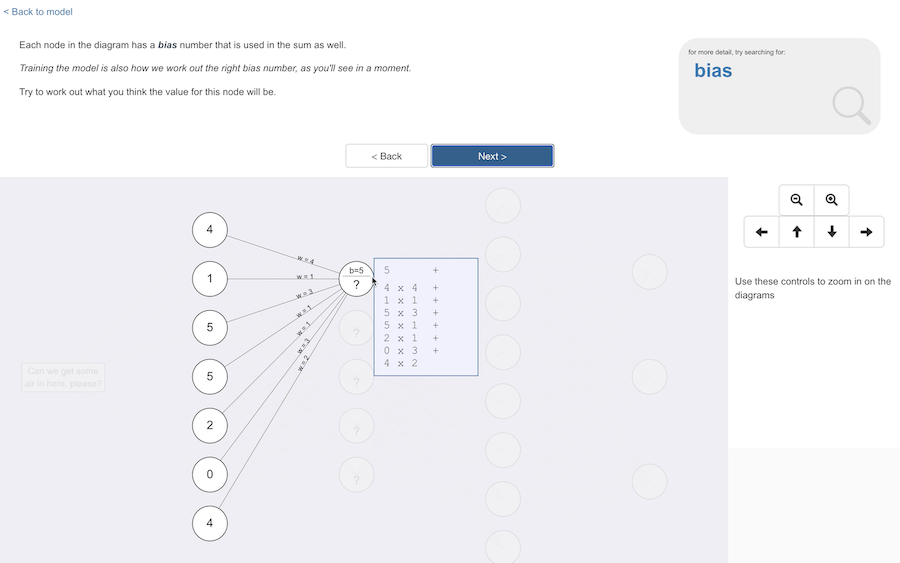Each node also has a number associated with it, that can be used in the sum too.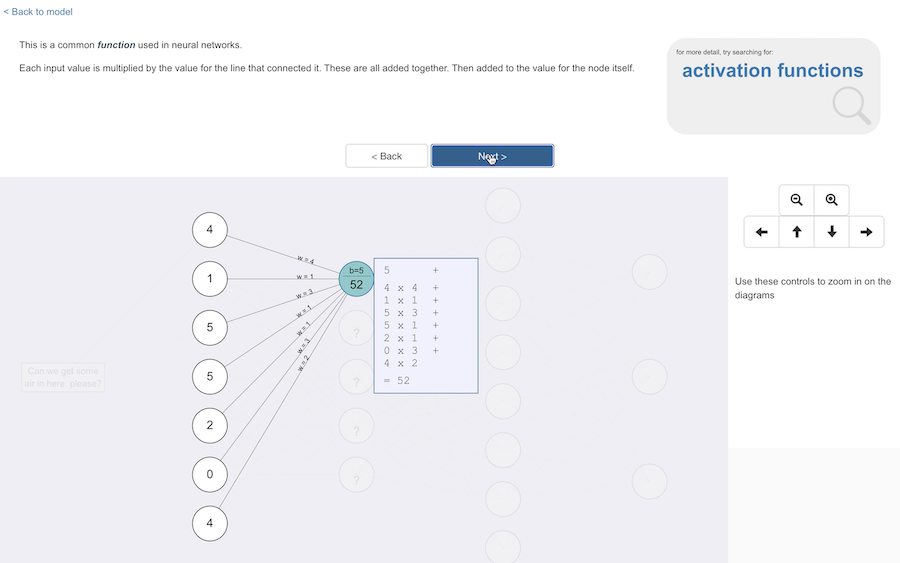This is a reasonable starting point to understand the sorts of functions that can be used: each input value is multiplied by weight for the line that connected it, and all of these are added together with a bias value for the node.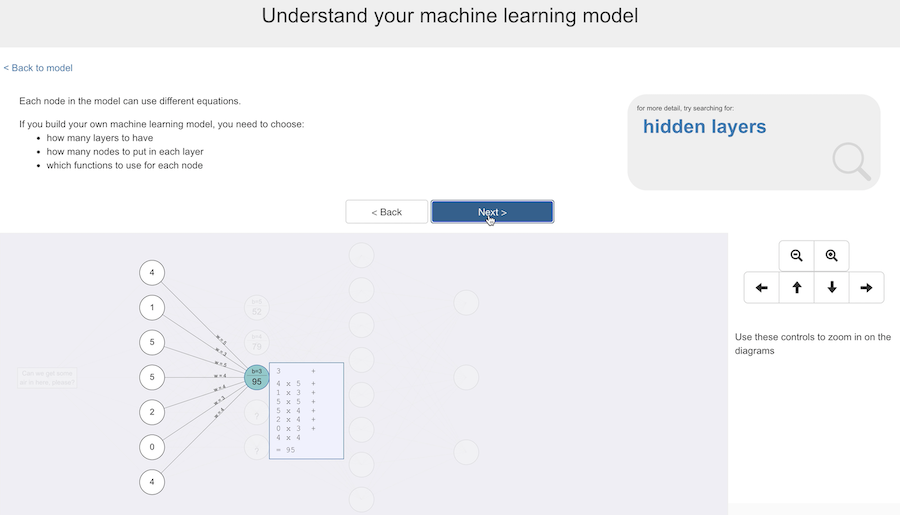The student is shown a few examples like this to get the idea.

And they’re reminded that if you build your own machine learning model, you need to choose how many layers to have, how many nodes to put in each layer, and what functions to use for each of the nodes (and that each node in the model can use different equations).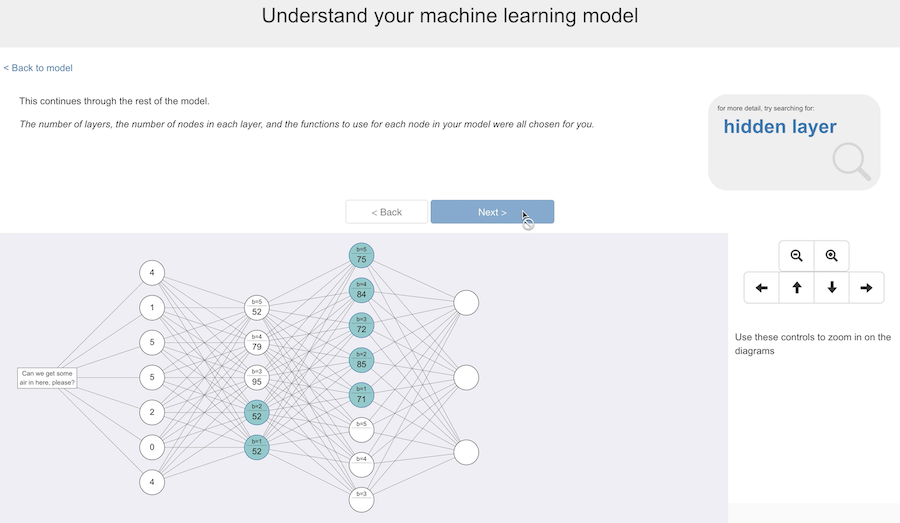This continues through the rest of the model until we reach the last layer.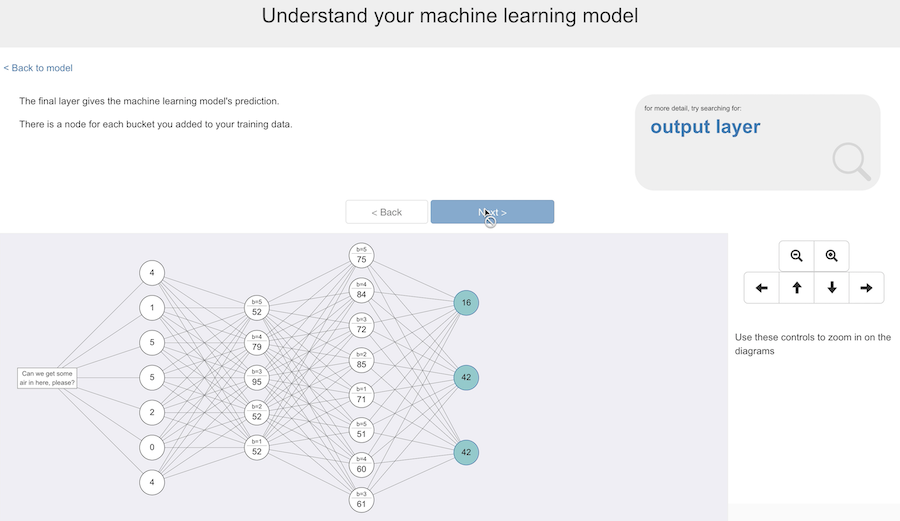The final layer has the output – this is the prediction the model has produced for this training input.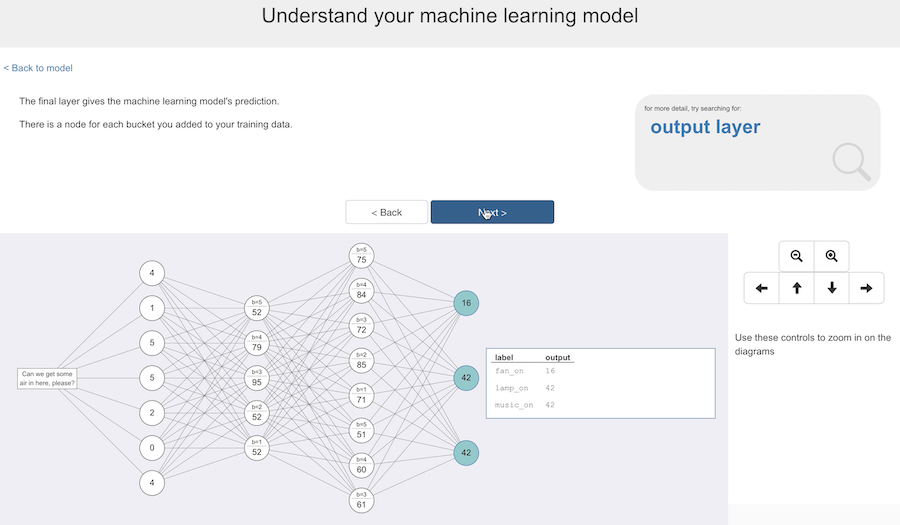This is customized to match their project. There are three nodes in the output layer in the diagram because there are three buckets of training data in this project.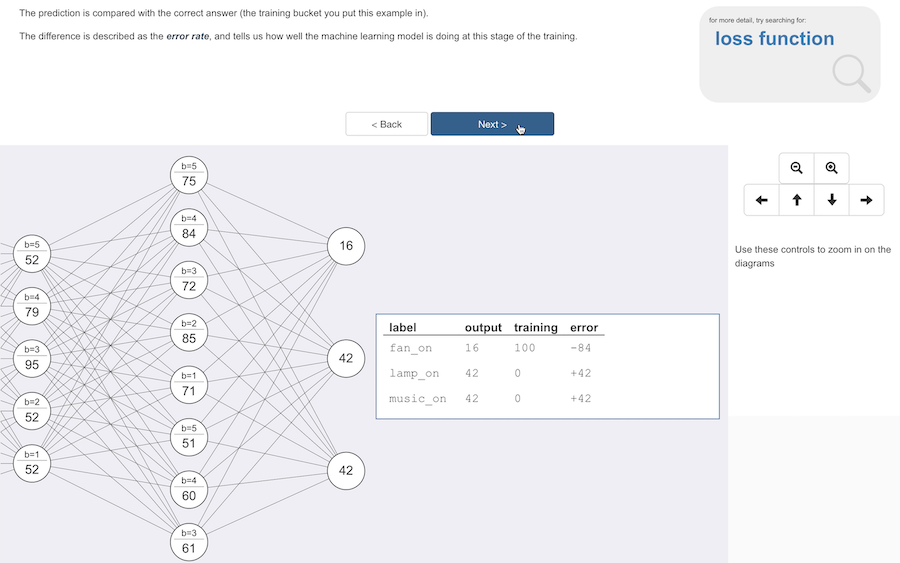The output from the model is compared with the correct answer.

This training example was taken from the fan_on bucket, so the correct answer would’ve been 100% for fan_on and 0% for everything else.

(This is more traditionally described as being a decimal number between 0 and 1, but in the rest of the site and in Scratch I refer to confidence as percentages, so I’m sticking with percentages to keep things consistent)

The comparison gives us the error rate for each output value.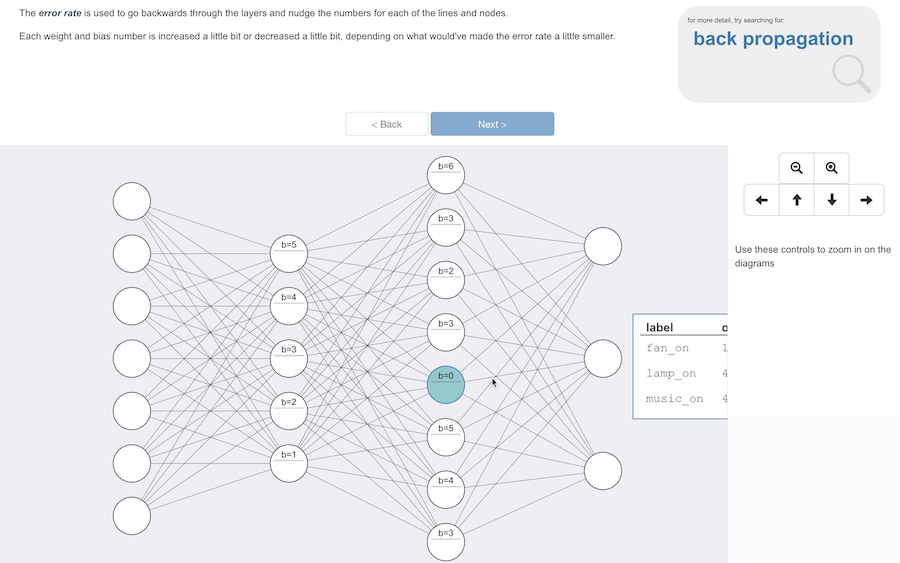This error rate is used to go backwards through the layers, and nudge the numbers for each of the lines and nodes.

Each weight and bias number is increased a little bit or decreased a little bit, depending on what would’ve made the error rate a little smaller.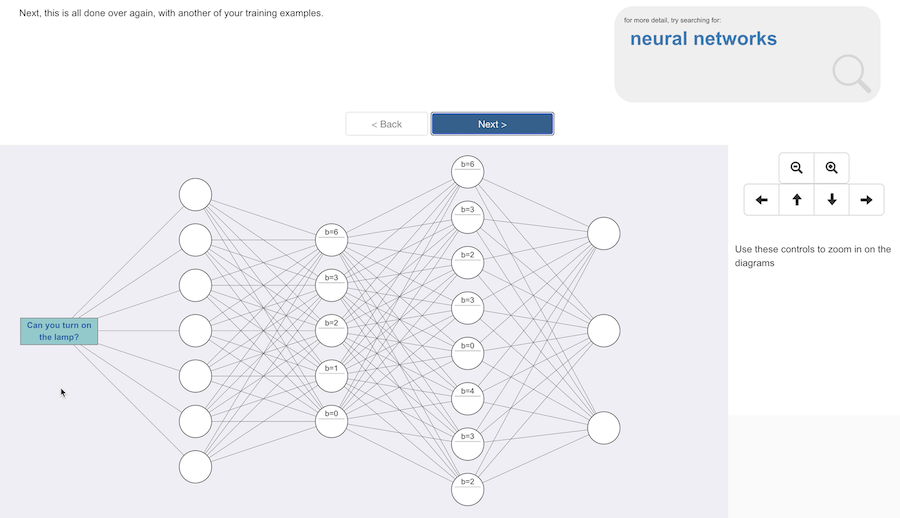Then we do this all over again, with another training example, but with the updated, nudged, values in the model.

Again, this is one of the training examples that the student will have put into their training bucket – something they will remember typing in.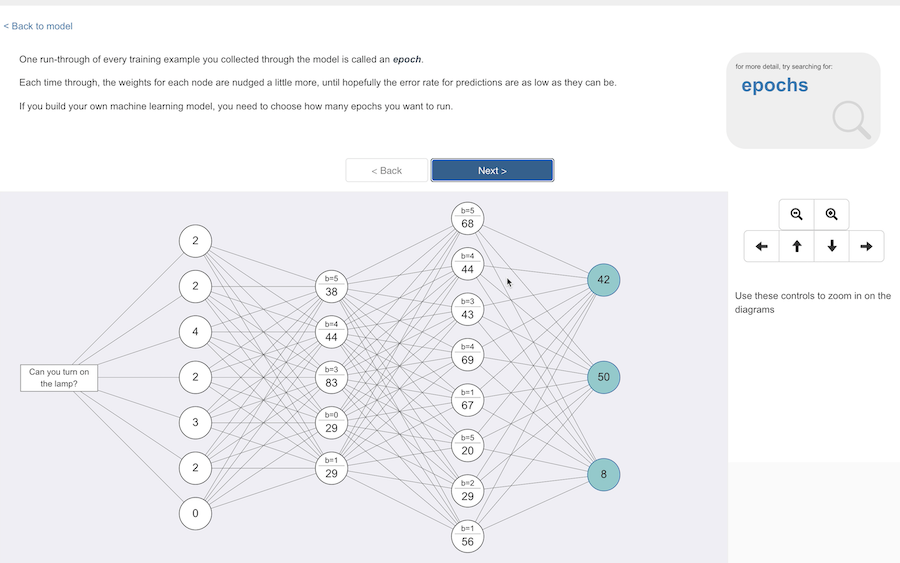I step the students through the first few of their training examples slowly, step by step.

And then once they get the idea, I start animating the rest of the training examples running through the model – turning each input text into numbers, calculating the values for the hidden layers until the final layer, comparing the output with the correct answer to calculate the error rate, and then using that to go back through the layers, adjusting the numbers associated with each of the lines and nodes.

Back and forwards, back and forwards, using their training examples to keep nudging the model values, until the predictions the model is making are good enough.

And they can go through all of the training examples more than once. The first time through, the predictions will likely be very bad, but by the time you’ve run every training example through, the model values will have been nudged so that the second run through can start from a much better place.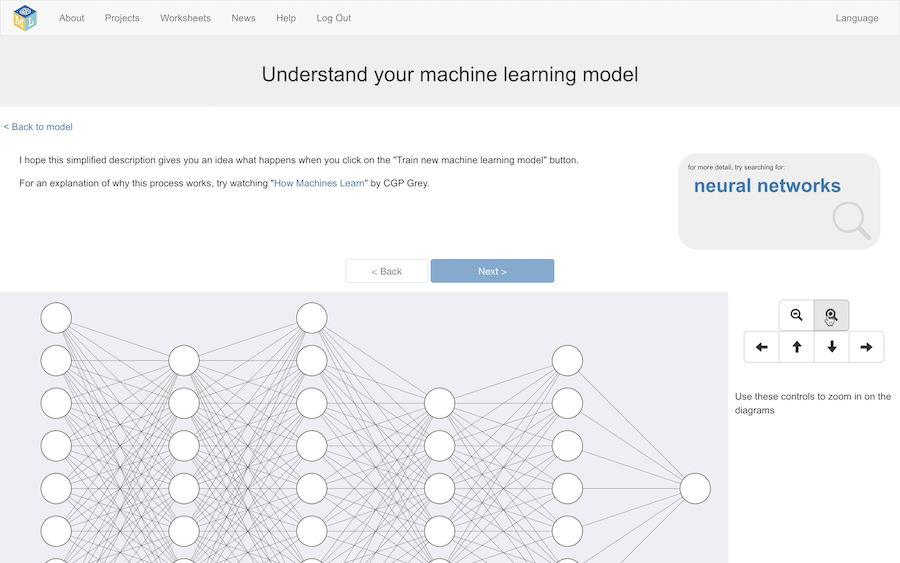And that’s it – a (hopefully!) simple introduction to the basic concepts for how a neural network is trained, that I customize based on the student’s own project.

I finish off with a link to a video that does a good job of explaining the intuition for why all of these endless tweaking of weight and bias values ends up producing something that can get the right answer.

### One Response to “Explaining ML with neural networks”

1. Mash says:

The ‘behind-the-scenes’ working of the ML projects was one missing link in your otherwise great portal. Kudos for adding it!!
I will go through it in more detail and leave my f/b

Thanks
Mash